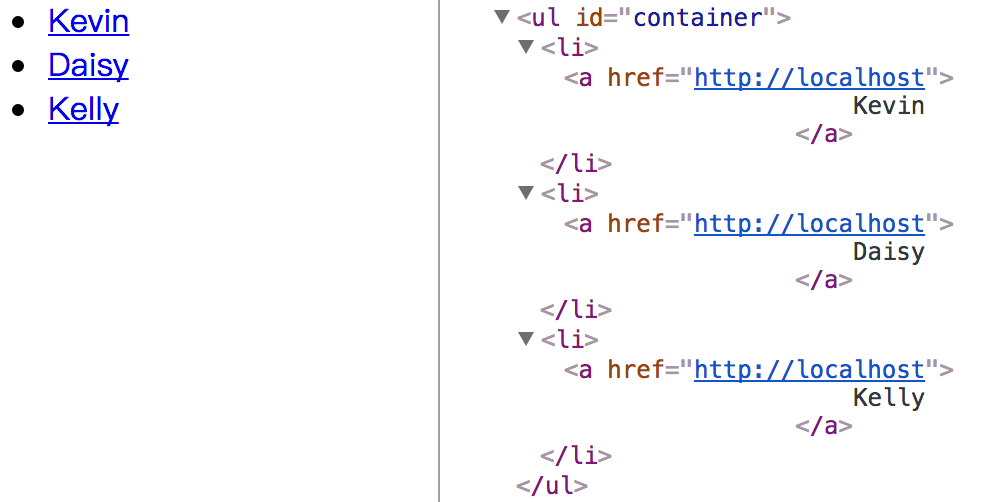# underscore 系列之实现一个模板引擎(上)

## 前言

underscore 提供了模板引擎的功能，举个例子：

```var tpl = "hello: <%= name %>";

var compiled = _.template(tpl);
compiled({name: 'Kevin'}); // "hello: Kevin"```

```<ul id="name_list"></ul>

<script type="text/html" id="user_tmpl">
<%for ( var i = 0; i < users.length; i++ ) { %>
<li>
<a href="<%=users[i].url%>">
<%=users[i].name%>
</a>
</li>
<% } %>
</script>```

JavaScript 文件中：

```var container = document.getElementById("name_list");

var data = {
users: [
{ "name": "Kevin", "url": "http://localhost" },
{ "name": "Daisy", "url": "http://localhost" },
{ "name": "Kelly", "url": "http://localhost" }
]
}
var precompile = _.template(document.getElementById("user_tmpl").innerHTML);
var html = precompile(data);

container.innerHTML = html;```## 实现思路

underscore 的 template 函数参考了 jQuery 的作者 John Resig 在 2008 年发表的一篇文章 JavaScript Micro-Templating，我们先从这篇文章的思路出发，思考一下如何写一个简单的模板引擎。

```<%for ( var i = 0; i < users.length; i++ ) { %>
<li>
<a href="<%=users[i].url%>">
<%=users[i].name%>
</a>
</li>
<% } %>```

John Resig 的思路是将这段代码转换为这样一段程序：

```// 模拟数据
var users = [{"name": "Kevin", "url": "http://localhost"}];

var p = [];
for (var i = 0; i < users.length; i++) {
p.push('<li><a href="');
p.push(users[i].url);
p.push('">');
p.push(users[i].name);
p.push('</a></li>');
}

// 最后 join 一下就可以得到最终拼接好的模板字符串
console.log(p.join('')) // <li><a href="http://localhost">Kevin</a></li>```

1. 将 `%>` 替换成 `p.push('`
2. 将 `<%` 替换成 `');`
3. 将 `<%=xxx%>` 替换成 `');p.push(xxx);p.push('`

``````<%for ( var i = 0; i < users.length; i++ ) { %>
<li>
<a href="<%=users[i].url%>">
<%=users[i].name%>
</a>
</li>
<% } %>
``````

``````');for ( var i = 0; i < users.length; i++ ) { p.push('
<li>
<a href="');p.push(users[i].url);p.push('">
');p.push(users[i].name);p.push('
</a>
</li>
'); } p.push('
``````

``````// 添加的首部代码
var p = []; p.push('

');for ( var i = 0; i < users.length; i++ ) { p.push('
<li>
<a href="');p.push(users[i].url);p.push('">
');p.push(users[i].name);p.push('
</a>
</li>
'); } p.push('

// 添加的尾部代码
');
``````

```var p = []; p.push('');
for ( var i = 0; i < users.length; i++ ) {
p.push('<li><a href="');
p.push(users[i].url);
p.push('">');
p.push(users[i].name);
p.push('</a></li>');
}
p.push('');```

## 第一版

```// 第一版
function tmpl(str, data) {
var str = document.getElementById(str).innerHTML;

var string = "var p = []; p.push('" +
str
.replace(/[\r\t\n]/g, "")
.replace(/<%=(.*?)%>/g, "');p.push(\$1);p.push('")
.replace(/<%/g, "');")
.replace(/%>/g,"p.push('")
+ "');"

eval(string)

return p.join('');
};```

HTML 文件：

```<script type="text/html" id="user_tmpl">
<%for ( var i = 0; i < users.length; i++ ) { %>
<li>
<a href="<%=users[i].url%>">
<%=users[i].name%>
</a>
</li>
<% } %>
</script>```

JavaScript 文件：

```var users = [
{ "name": "Byron", "url": "http://localhost" },
{ "name": "Casper", "url": "http://localhost" },
{ "name": "Frank", "url": "http://localhost" }
]
tmpl("user_tmpl", users)```

## Function

Function 构造函数创建一个新的 Function 对象。 在 JavaScript 中, 每个函数实际上都是一个 Function 对象。

`new Function ([arg1[, arg2[, ...argN]],] functionBody)`

arg1, arg2, … argN 表示函数用到的参数，functionBody 表示一个含有包括函数定义的 JavaScript 语句的字符串。

```var adder = new Function("a", "b", "return a + b");

## 第二版

```// 第二版
function tmpl(str, data) {
var str = document.getElementById(str).innerHTML;

var fn = new Function("obj",

"var p = []; p.push('" +

str
.replace(/[\r\t\n]/g, "")
.replace(/<%=(.*?)%>/g, "');p.push(\$1);p.push('")
.replace(/<%/g, "');")
.replace(/%>/g,"p.push('")
+ "');return p.join('');");

return fn(data);
};```

## with

```var data = {
status: 200,
name: 'kevin',
friends: [...]
}```

with 语句可以扩展一个语句的作用域链(scope chain)。当需要多次访问一个对象的时候，可以使用 with 做简化。比如：

```var hostName = location.hostname;
var url = location.href;

// 使用 with
with(location){
var hostname = hostname;
var url = href;
}```
```function Person(){
this.name = 'Kevin';
this.age = '18';
}

var person = new Person();

with(person) {
console.log('my name is ' + name + ', age is ' + age + '.')
}
// my name is Kevin, age is 18.```

## 第三版

```// 第三版
function tmpl(str, data) {
var str = document.getElementById(str).innerHTML;

var fn = new Function("obj",

// 其实就是这里多添加了一句 with(obj){...}
"var p = []; with(obj){p.push('" +

str
.replace(/[\r\t\n]/g, "")
.replace(/<%=(.*?)%>/g, "');p.push(\$1);p.push('")
.replace(/<%/g, "');")
.replace(/%>/g,"p.push('")
+ "');}return p.join('');");

return fn(data);
};```

## 第四版

```// 第四版
function tmpl(str, data) {
var str = document.getElementById(str).innerHTML;

var fn = new Function("obj",

"var p = []; with(obj){p.push('" +

str
.replace(/[\r\t\n]/g, "")
.replace(/<%=(.*?)%>/g, "');p.push(\$1);p.push('")
.replace(/<%/g, "');")
.replace(/%>/g,"p.push('")
+ "');}return p.join('');");

var template = function(data) {
return fn.call(this, data)
}
return template;
};

// 使用时
var compiled = tmpl("user_tmpl");
results.innerHTML = compiled(data);```

## 下期预告